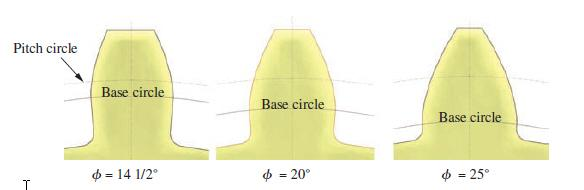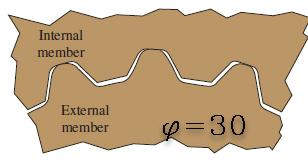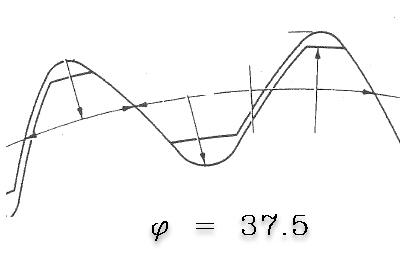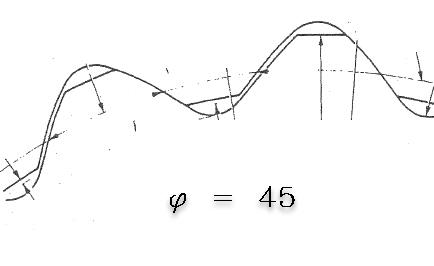# Involute profile in splines (1)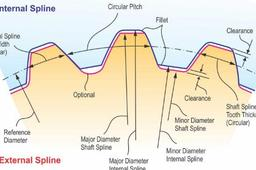This is a short description of how involute profiles are used in splines like DIN 5480, DIN 5482, ISO 4256, and ANSI 92.1

1. ### Step 1: Generation of an involute profile

A curve that is obtained by attaching a string that is imaginary and then winding and unwinding it tautly on the curve given is called involute in differential geometry.

The involutes of the different curves as a Circle, a Catenary, a Deltoid, a Parabola, an Ellipse, and so on.

The involutes used in gearing or in splines connections are involutes of circles. Named Base Circle. See the figure below. (1)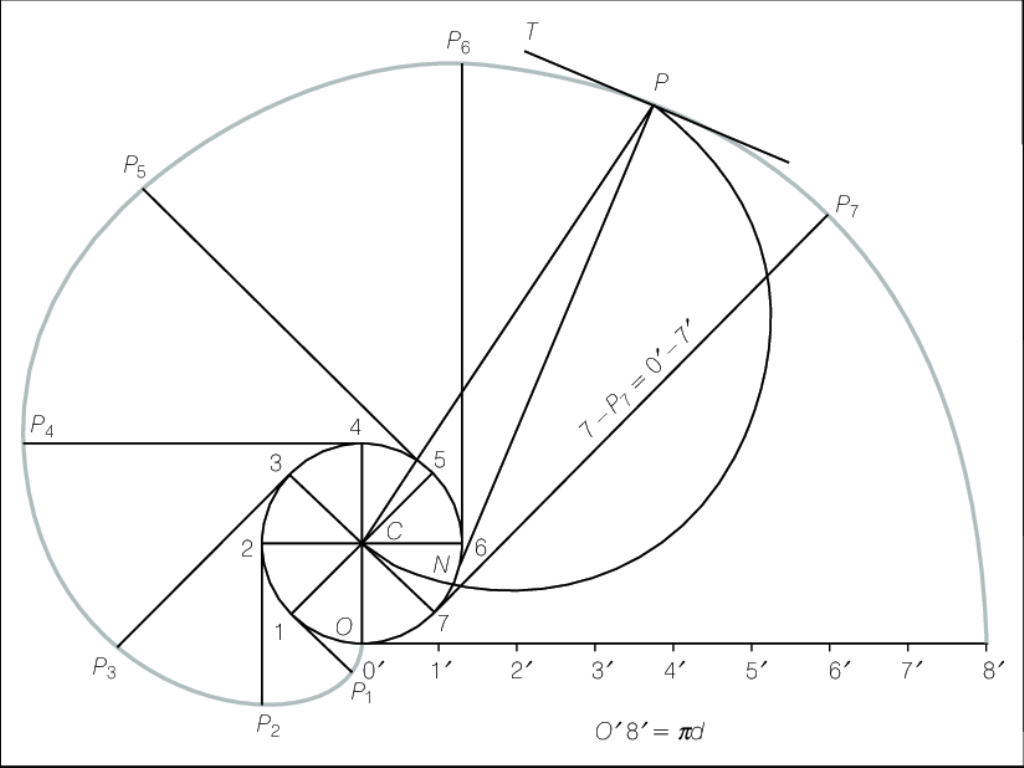2. ### Step 2: Involute in gears and splines

In splines or spur gears, a fraction of the profiles is an involute of a circle.

See the figure below. (2)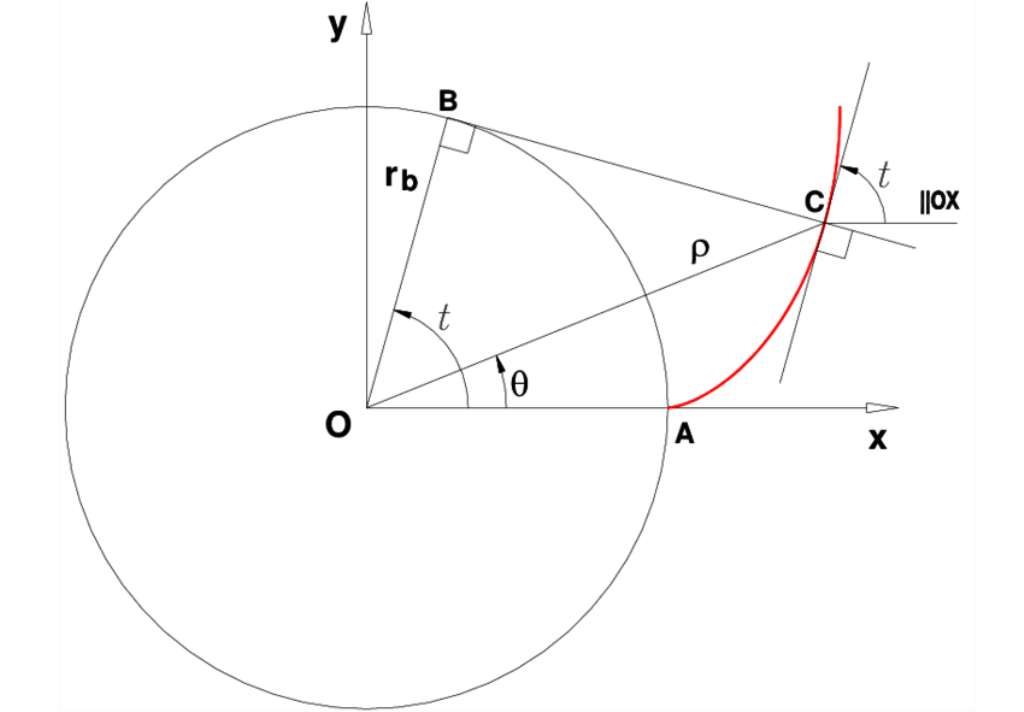3. ### Step 3: Internal and external splines

Involute Spline is a spline having teeth with involute profiles. They can be external or internal. External in the shaft and internal in the hub.

See the figure below. (3)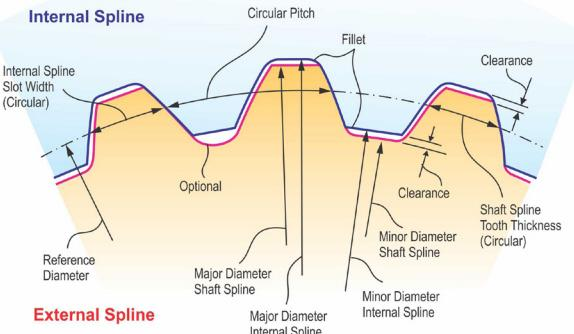4. ### Step 4: Diametral pitch and Module

This applies to DIN 5480, DIN 5482, ISO 4156, ANSI B92.2M, ANSI B92.1, and similar splines

The imperial system uses DIAMETRAL PITCH to characterize teeth size. Diametral pitch is always in inches.

Pd = N/D = number of teeth to inches of pitch diameter

N: number of teeth

D: pitch diameter in inches

The metric system uses MODULE to characterize teeth size. Module pitch is always in millimeters.

Module m = D/N = mm of pitch diameter per tooth

N : number of teeth

D : pitch diameter in mm

A gear with 10 teeth and 1.000 inch pitch diameter

10 diametral pitch = 10 / 1.000in

The same gear in metric:

2.54 module = 25.4mm / 10

m = 25.4/Pd  Pd = 25.4/m

5. ### Step 5: Pitch circle

Pitch Circle is the reference circle from which all circular spline tooth dimensions are constructed. Its diameter is determined as the ratio of the number of teeth to the diametral pitch.

Pitch Diameter, D The diameter of the pitch circle.

See the figure below. (4)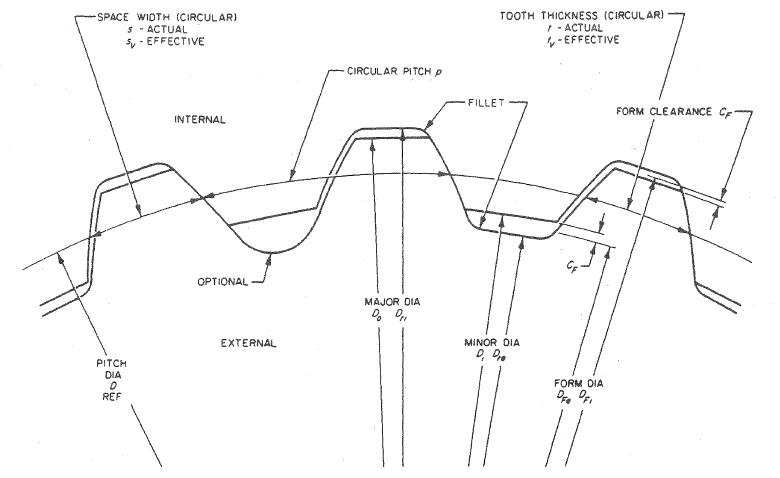6. ### Step 6: Pressure angle

The pressure angle is the angle between the tangent to the pitch circles and the line drawn normal (perpendicular) to the surface of the gear tooth

See the figure below. (5)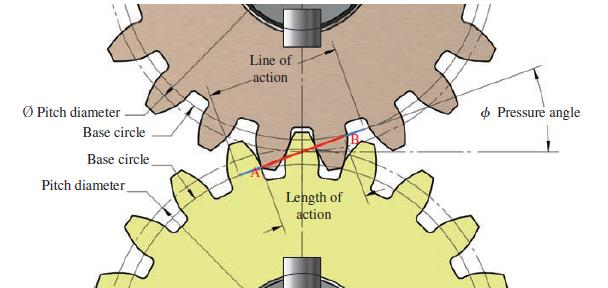7. ### Step 7: Pressure angle examples

Illustration bellow of how the shape of gear teeth change as the pressure angle, changes. (6)  Involute splines are typically made with pressure angles of 30°, 37.5°, or 45°.

The DIN 5480 and DIN 5482 series of standards is limited to splines with a pressure angle of 30°, since pressure angles of 37.5° and 45° are covered by ISO 4156

ANSI B92.1 covers pressure angles of 30, 37.5° and 45°